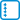## Number of fixed points in a random permuation

Consider a random permutation of $$n$$ elements. Determine the expected value of the number of fixed points of such a permutation (i.e. the number of elements $$i$$ such that $$\pi(i) = i$$, if $$\pi$$ is the random permutation).

• #### Solution

We introduce an indicator variable $$I_i$$ indicatinng that $$i$$ is a fixed point of the permutation.

We have $$EI_i=P(\pi(i)=i)=\frac{(n-1)!}{n!}=\frac{1}{n}$$.

From there, $$EX=EI_1+…+EI_n=1$$.